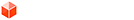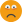## Hide/Show only every Nth Copied Instance?

### Hide/Show only every Nth Copied Instance?

I hope this hasn't been covered somewhere else, but I can't find it anywhere

To keep it simple, I have a variable that is set by the user (say 3), and I want to hide every COPY multiple of that number, including the original.

So, I'm hiding COPY 0.0, COPY 3.0, COPY 6.0, etc.

BT
0

bthomas3

### Re: Hide/Show only every Nth Copied Instance?

I suppose there's a math formula for this, I just don't know it. Otherwise, if you don't plan on having many copies, you can do a really long IF statement to hide copy 3, 6, 9, etc). Maybe add another variable to test copy# so that you can easily change what Nth number you want hidden.
Thinking out loud, I'm thinking having a variable to calculate the total copies/# (like 3)='A', and with that number you'll get the total amount of copies you need hidden. And then somehow get every 3x# up to 'A' hidden...
0

quantj

### Re: Hide/Show only every Nth Copied Instance?

Usage: Select any group/component, right click, and select Hide Every nth Instance option.
0

Anton_S
PluginStore Author### Re: Hide/Show only every Nth Copied Instance?

Anton_S wrote:Usage: Select any group/component, right click, and select Hide Every nth Instance option.

Excellent script, thank you, but not ideal for this application. I need something in Dynamic Components so that the object (say a white picket fence) can be scaled to length AND the user can specify the interval of hidden components.

The flip side to this is a second, overlapping set of pickets (say, blue), that show up where the others were removed. This formula is easy using COPY*Multiplier*distance, but hiding the white ones that the blue ones replace is where I'm stuck.

Thanks again

BT
0

bthomas3

### Re: Hide/Show only every Nth Copied Instance?

quantj wrote:I suppose there's a math formula for this, I just don't know it. Otherwise, if you don't plan on having many copies, you can do a really long IF statement to hide copy 3, 6, 9, etc). Maybe add another variable to test copy# so that you can easily change what Nth number you want hidden.
Thinking out loud, I'm thinking having a variable to calculate the total copies/# (like 3)='A', and with that number you'll get the total amount of copies you need hidden. And then somehow get every 3x# up to 'A' hidden...

Ok, I was trying to avoid If/Then statements, but I got it to work with your suggestion, so time to move on. Here's what I have:

Variable (var) = 2 or 3

For the "white pickets" hidden every 2 or 3 instances:
=IF(COPY=0,0,IF(COPY=var*1,0,IF(COPY=var*2,0,IF(COPY=var*3,1,0))))

For the "blue pickets" shown every 2 or 3 instances:
=IF(COPY=0,0,IF(COPY=var*1,0,IF(COPY=var*2,0,IF(COPY=var*3,0,1))))

Thanks for your help, everyone. If there's a more elegant solution, I'm all ears. Otherwise, this will do. Thanks again!

BT
0

bthomas3

### Re: Hide/Show only every Nth Copied Instance?

only variable that acts as a counter is copy
so need to make an expression using this when comparing to other variables

know each object is copy+1

also its a multiple of the "missing rate" (num)

however sometimes its a perfect division other times not

so need to make a formula which gives the decimal part of the division

=(copy+1)/num-int((copy+1)/num)

then use this as if statement
1

pcmoor

### Re: Hide/Show only every Nth Copied Instance?

I think I kinda got something going:

Nth=3(or any other number)

Formula A: =copy/3
example: 8/3 = 2.6667
Formula B: =INT(copy/3)
example: 8/3 = 2
Formula C: =Formula A - Formula B

Hidden: =IF(Formula C = 0, 0, 1)

I worked once for my example, but when i changed the number of copies, it didn't auto redraw to redo the formula.
Hopefully someone here can finish this with native tools, it's a puzzle I'd like to see solved without plug-ins
0

quantj

### Re: Hide/Show only every Nth Copied Instance?

pcmoor wrote:only variable that acts as a counter is copy
so need to make an expression using this when comparing to other variables

know each object is copy+1

also its a multiple of the "missing rate" (num)

however sometimes its a perfect division other times not

so need to make a formula which gives the decimal part of the division

=(copy+1)/num-int((copy+1)/num)

then use this as if statement

Sorry pcmoor, my math stills arent tha advanced, that went over my head.Tried out your model (after writing my post), yours works and can update (which i couldn't figure out)

EDIT:
I get it! after i stared at the INT i realized we were on the same track, i think just seeing all the variables put into one formula confused me
0

quantj

### Re: Hide/Show only every Nth Copied Instance?

You should be using a modulo operation for this. A modulo operation will give the "remainder" of integer division. SketchUp doesn't provide a mod function for DCs, so you'll have to create it as such:
Code: Select all
`a - (n * FLOOR(a/n))`
where a is the index and you want to hide every N.

For example, if you have
Code: Select all
`HIDDEN=COPY - (3 * FLOOR(COPY/3))==0`
, you'll get:
Code: Select all
`HIDDEN, VISIBLE, VISIBLE, HIDDEN`
.

You can change the compared value (in the above example, 0) to be the "offset", but it must be less than n.

For example, if you have
Code: Select all
`HIDDEN=COPY - (3 * FLOOR(COPY/3))==2`
, you'll get:
Code: Select all
`VISIBLE, VISIBLE, HIDDEN, VISIBLE, ...`
.
0danielbowring

### Re: Hide/Show only every Nth Copied Instance?

Actually Ruby does have a modulo function. It's this symbol: %.

5%3 = 2
6%3 = 0
etc...

The script at third post uses the modulo technique to hide every nth instance.
0

Anton_S
PluginStore Author### Re: Hide/Show only every Nth Copied Instance?

Yes, ruby does have a modulo operator - I said Dynamic Components doesn't provide a modulus function.
0danielbowring